# KSEEB Solutions for Class 9 Maths Chapter 15 Probability Ex 15.1

KSEEB Solutions for Class 9 Maths Chapter 15 Probability Ex 15.1 are part of KSEEB Solutions for Class 9 Maths. Here we have given Karnataka Board Class 9 Maths Chapter 15 Probability Exercise 15.1.

## Karnataka Board Class 9 Maths Chapter 15 Probability Ex 15.1.

Question 1.
In a cricket match, a batswoman hits a boundary 6 times out of 30 balls she plays. Find the probability that she did not hit a boundary.
Solution:
Total number of hittings of ball = 30
∴ n(S) = 30.
Total number of boundary = 6
Total number of No boundaries = 30 – 6 = 24
∴ n(E) = 24
Probability of No boundary hits =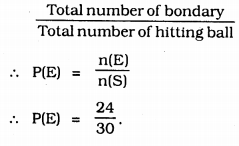Question 2.
1500 families with 2 children were selected randomly, and the following data were recorded :

 Number of girls in a family 2 1 0 Number of families 475 814 211

Compute the probability of a family, chosen at random, having
(i) 2 girls
(ii) 1 girl,
(iii) No girl
Also check whether the sum of these probabilities is 1.
Solution:
Total number of families =1500
(i) Family with 2 girls = 475
∴ P1(Family with 2 girls)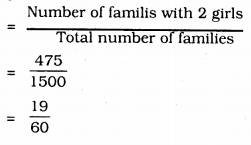(ii) Family with 1 girl = 814
∴ P2 (Family with 1 girl)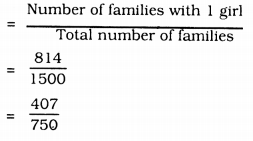(iii) Family without girls = 211
∴ P3 (Family without girls)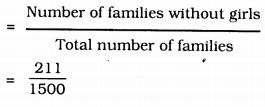Sum of probabilities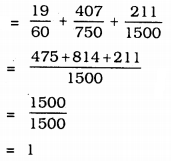∴ Sum of probabilities is 1.

Question 3.
Refer to Example 5, Section 14.4, Chapter 14. Find the probability that a student of the class was born in August.
Solution:
Total number of students in IX class = 40
∴ n(S) = 40
Number of students born in August = 6
∴ n(E) = 6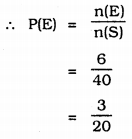Question 4.
Three coins are tossed simultaneously 200 times with the following frequencies of different outcomes :

 Outcome 3 Heads 2 Heads 1 Head No head Frequency 23 72 77 28

If the three coins are simultaneously tossed again, compute the probability of 2 heads coming up.
Solution:
Total number of events tossing 3 coins simultaneously = 200
∴ n(S) = 200
Frequency in which two heds up = 72
∴ n(E) = 72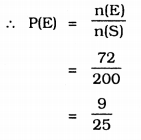Question 5.
An organisation selected 2400 families at random and surveyed them to determine a relationship between income level and the number of vehicles in a family. The information gathered is listed in the table below :

 Monthly Income (in Rs.) Vehicles per family 0 1 2 Above 2 Less than 7000 10 160 25 0 7,000 – 10,000 0 305 27 2 10,000- 13,000 1 535 29 1 13,000- 16,000 2 469 59 25 16,000 or more 1 579 82 88

Suppose a family is chosen. Find the probability that the family chosen is
(i) earning Rs. 10000 – 13000 per month and owning exactly 2 vehicles.
(ii) earning Rs. 16000 or more per month and owning exactly 1 vehicle.
(iii) earning less than Rs. 7000 per month and does not own any vehicle.
(iv) earning Rs. 13000 – 16000 per month and owning more than 2 vehicles.
(v) owning not more than 1 vehicle.
Solution:
Total number of families surveyed = 2400
∴ n(S) = 2400
(i) earning Rs. 10000 – 13000 per month and owning exactly 2 vehicles = 29
∴ n(A) = 29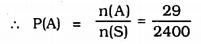(ii) earning Rs. 16000 or more per month and owning exactly 1 vehicle = 579
∴ n(B) = 579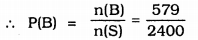(iii) earning less than Rs. 7000 per month and does not own any vehicle =10
∴ n(C) = 10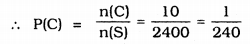(iv) earning Rs. 13000 – 16000 per month and owning more than 2 vehicles = 25
∴ n(D) = 25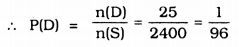(v) owning not more than 1 vehicle 10 + 0 + 1 + 2 + 1 + 160 + 305 + 535 + 469 + 579 = 2062
∴ n(E) = 2062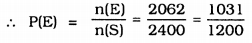Question 6.
Refer to Table 14.7, Chapter 14.
(i) Find the probability that a student obtained less than 20% in the Mathematics test.
(ii) Find the probability that a student obtained marks 60 or above.
Solution:
(i) Number of students obtained less than 20% in the Mathematics test = 7
n(A) = 7
Total number of students = 90
n(S) = 90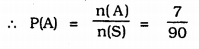(ii) Number of students obtained marks 60 or above
= 15 + 8 = 23 ∴ n(B) = 23
Total Number of students = 90
n(S) = 90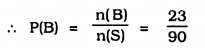Question 7.
To know the opinion of the students about the subject statistics, a survey of 200 students was conducted. The data is recorded in the following table:

 Opinion No. of students like dislike 135 65

Find the probability that a student chosen at random.
(i) likes statistics,
(ii) does not like it.
Solution:
Total number of students = 135 + 65
= 200
n(S) = 200
(i) Number of students who like Statistics
= 135
n(A) = 135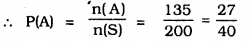(ii) Number of students who does not like Statistics = 65
n(B) = 65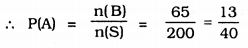Question 8.
Refer to Q. 2, Exercise 14.2. What is the empirical probability that an engineer lives :
(i) less than 7 km from her place of work ?
(ii) more than or equal to 7 km from her place of work ?
(iii) within $$\frac{1}{2}$$ km from her place of work.
Solution:
Referring to Q.2 Exercise 14.2
(i) Total Number of engineers = 40
n(S) = 40
Number of engineers living less than 7 km from her place of work = 9
n(A) = 9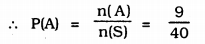(ii) Number of engineers living more than or equal to 7 km from her place of work.
= 40 – 9 = 31
n(B) = 31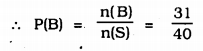(iii) Number of engineers living within $$\frac{1}{2}$$ Km from her place of work = 0
n(C) = 0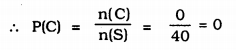Question 9.
Activity: Note the frequency of two-wheelers, three-wheelers and four- wheelers going past during a time interval, in front of your school gate. Find the probability that any one vehicle out of the total vehicles you have observed is a two-wheeler.

Question 10.
Activity: Ask all the students in your class to write a 3-digit number. Choose any student from the room at random. What is the probability that the number written by her/him is divisible by 3? Remember that a number is divisible by 3, if the sum of its digits is divisible by 3.

Question 11.
Eleven bags of wheat flour, each marked 5 kg, actually contained the following weights of flour (in kg.)
4.97 5.05 5.08 5.03 5.00 5.06
5.08 4.98 5.04 5.07 5.00
Find the probability that any of these bags chosen at random contains more than 5 kg of flour.
Solution:
Total number of bags of wheat flour = 11
n(S) = 11
Number of bags which have flour more than 5 kg. = 7
n(A) = 0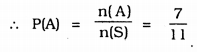Question 12.
In Q. 5, Exercise 14.2, you were asked to prepare a frequency distribution table, regarding the concentration of sulphur dioxide in the air in parts per million of a certain city for 30 days. Using this table, find the probability of the concentration of sulphur dioxide in the interval 0.12- 0.16 on any of these days.
Solution:
In Q.5, Exercixe 14.2 of the Text Book,
Number of days recorded = 30
n(S) = 30
Concentration of Sulphur Dioxide (SO2) in the interval 0.12 – 0.16 = 2
n(A) = 2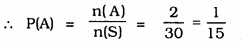Question 13.
In Q.1, Exercise 14.2, you were asked to prepare a frequency distribution table regarding the blood groups of 30 students of a class. Use this table to determine the probability that a student of this class, selected at random, has blood group AB.
Solution:
In Q. 1, Exercise 14.2 of the Text Book,
Total Number of students = 30
n(S) = 30
Number of students having Blood group AB = 3
n(A) = 3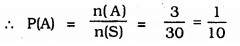We hope the KSEEB Solutions for Class 9 Maths Chapter 15 Probability Ex 15.1 help you. If you have any query regarding Karnataka Board Class 9 Maths Chapter 15 Probability Exercise 15.1, drop a comment below and we will get back to you at the earliest.